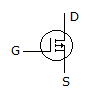# Electronics and Communication Engineering - Analog Electronics

11.

When narrow current pulses drive a high Q resonant circuit the voltage across the circuit looks like

 A. narrow pulses B. half sine wave C. almost sine wave D. either (a) or (b)

Explanation:

No answer description available for this question. Let us discuss.

12.

The multivibrator circuit that has a typical application of converting sinusoidal input into a square wave output is

 A. emitter coupled binary B. Schmitt trigger C. monoshot multivibrator D. free-running multivibrator

Explanation:

No answer description available for this question. Let us discuss.

13.

Symbol shown in figure represents aA. P-channel enhancement MOSFET B. N-channel DEMOSFET C. UJT with P-type base D. UJT with N-type base

Explanation:

No answer description available for this question. Let us discuss.

14.

The feedback resistance rd in small signal model of MOSFET is of the order of

 A. 1000 MΩ B. 1 MΩ C. 1 KΩ D. 100 Ω

Explanation:

No answer description available for this question. Let us discuss.

15.

Which amplifier has a voltage gain of less than 1?

 A. Common emitter B. Common collector C. Common base D. Both CB and CC